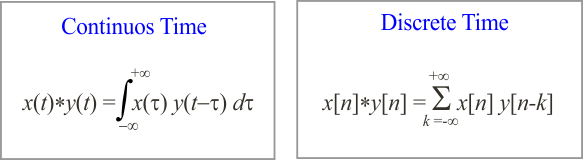# Convolution

## The Convolution

In the areas of Digital Signal Processing (DSP) and Communications the operation of convolution plays a very important role because it can be used to find the output of a Linear Time-Invariant system (LTI) and simplify several operations. The discrete convolution is computed between two sequences as shown below. The continuous convolution is computed between two continuous signals.